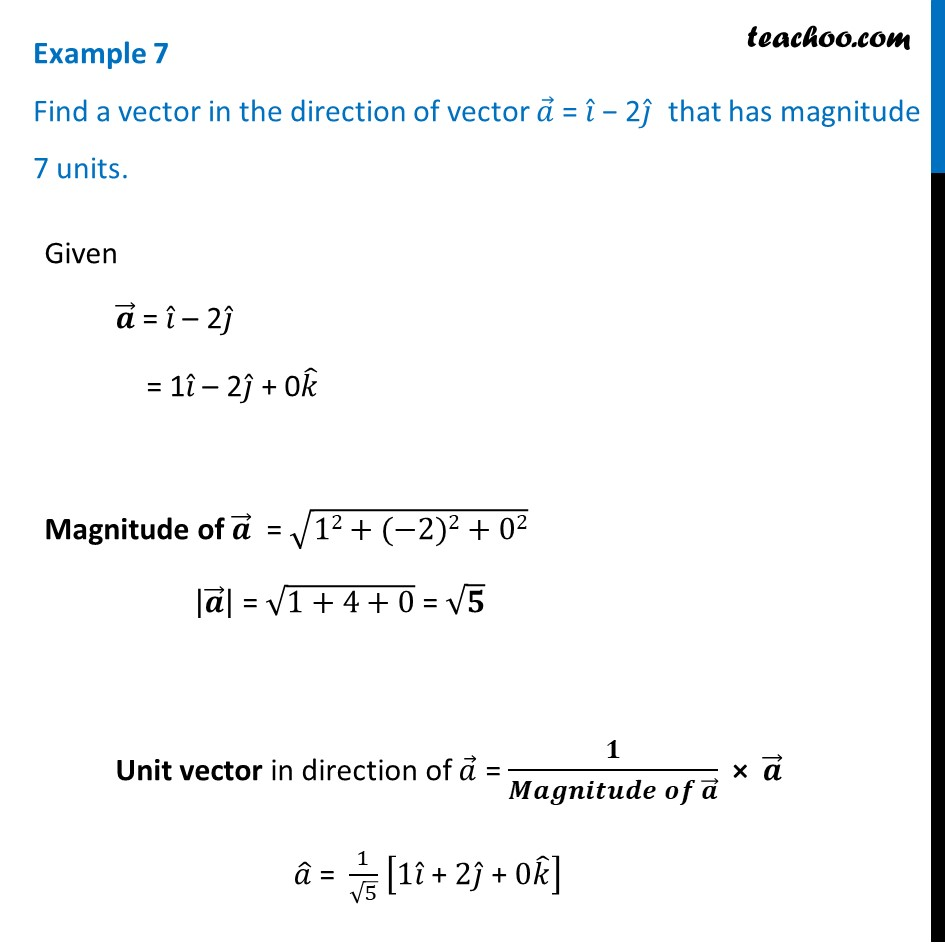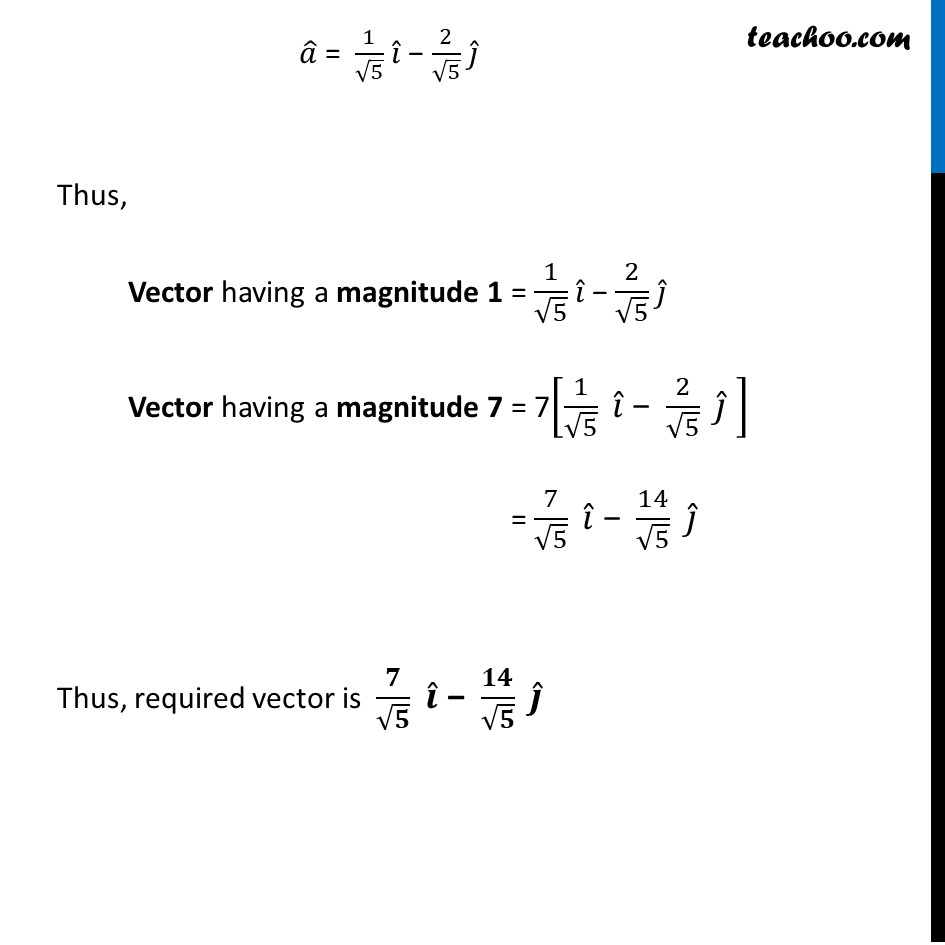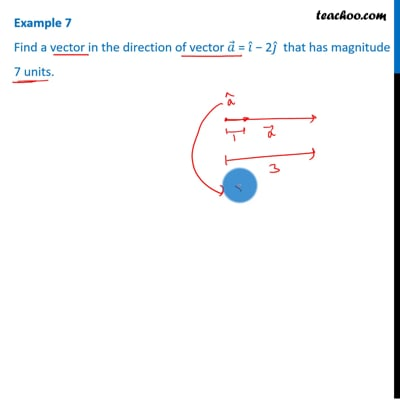Examples

Chapter 10 Class 12 Vector Algebra
Serial order wiseThis video is only available for Teachoo black users

Solve all your doubts with Teachoo Black (new monthly pack available now!)

### Transcript

Example 7 Find a vector in the direction of vector 𝑎 ⃗ = 𝑖 ̂ − 2𝑗 ̂ that has magnitude 7 units. Given 𝒂 ⃗ = 𝑖 ̂ – 2𝑗 ̂ = 1𝑖 ̂ – 2𝑗 ̂ + 0𝑘 ̂ Magnitude of 𝒂 ⃗ = √(12+(−2)2+02) |𝒂 ⃗ | = √(1+4+0) = √𝟓 Unit vector in direction of 𝑎 ⃗ = 𝟏/(𝑴𝒂𝒈𝒏𝒊𝒕𝒖𝒅𝒆 𝒐𝒇 𝒂 ⃗ ) × 𝒂 ⃗ 𝑎 ̂ = 1/√5 ["1" 𝑖 ̂" + " 2𝑗 ̂" + " 0𝑘 ̂ ] 𝑎 ̂ = 1/√5 𝑖 ̂ − 2/√5 𝑗 ̂ Thus, Vector having a magnitude 1 = 1/√5 𝑖 ̂ − 2/√5 𝑗 ̂ Vector having a magnitude 7 = 7[1/√5 " " 𝑖 ̂" − " 2/√5 " " 𝑗 ̂" " ] = 7/√5 " " 𝑖 ̂" − " 14/√5 " " 𝑗 ̂ Thus, required vector is 𝟕/√𝟓 " " 𝒊 ̂" − " 𝟏𝟒/√𝟓 " " 𝒋 ̂